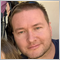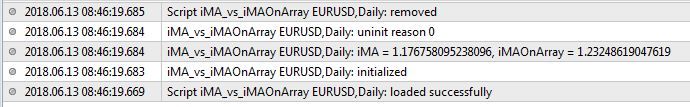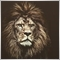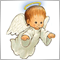# iMA vs iMAOnArray6414

I have some problems with understanding iMAOnArray for MQL4. Below is script's code, where I am trying to compare results between iMa and iMAOnArray. In my opinion, if I prepare array with prices and use iMAOnArray on the basis of this array, the result must be the same as I just use iMA. Probably somewhere is a logical mistake in my code. If this topic was discussed somewhere before, please give mi a link. Thx.

```#property strict
void OnStart()
{
//---
int period=21;
//--- 1
double value_iMA=iMA(_Symbol,PERIOD_D1,period,0,MODE_SMA,PRICE_CLOSE,7);
//--- 2
double closeArray;
for(int i=0; i<100; i++)
closeArray[i]=iClose(_Symbol,PERIOD_D1,i);
double value_iMAOnArray=iMAOnArray(closeArray,0,period,0,MODE_SMA,7);
//---
Print("iMA = ",value_iMA,", iMAOnArray = ",value_iMAOnArray);
}```

Result:9850

Maxim Khrolenko:

I have some problems with understanding iMAOnArray for MQL4. Below is script's code, where I am trying to compare results between iMa and iMAOnArray. In my opinion, if I prepare array with prices and use iMAOnArray on the basis of this array, the result must be the same as I just use iMA. Probably somewhere is a logical mistake in my code. If this topic was discussed somewhere before, please give mi a link. Thx.

Result:

Set the array as series

```//---
int period=21;
//--- 1
double value_iMA=iMA(_Symbol,PERIOD_D1,period,0,MODE_SMA,PRICE_CLOSE,7);
//--- 2
double closeArray;
ArraySetAsSeries(closeArray,true);
for(int i=0; i<100; i++)
closeArray[i]=iClose(_Symbol,PERIOD_D1,i);
double value_iMAOnArray=iMAOnArray(closeArray,0,period,0,MODE_SMA,7);
//---
Print("iMA = ",value_iMA,", iMAOnArray = ",value_iMAOnArray);```3051

Maxim Khrolenko:

I have some problems with understanding iMAOnArray for MQL4. Below is script's code, where I am trying to compare results between iMa and iMAOnArray. In my opinion, if I prepare array with prices and use iMAOnArray on the basis of this array, the result must be the same as I just use iMA. Probably somewhere is a logical mistake in my code. If this topic was discussed somewhere before, please give mi a link. Thx.

Result:

The problem is in arrays direction. Your array "closeArray" isn't "AsSeries". Try this part of code instead of your part.

```for(int i=0; i<100; i++)
closeArray[99-i]=iClose(_Symbol,PERIOD_D1,i);
double value_iMAOnArray=iMAOnArray(closeArray,0,period,0,MODE_SMA,99-7);```6414

Thanks everyone. Really, I forgot about direction. ArraySetAsSeries() fixed the problem. :)